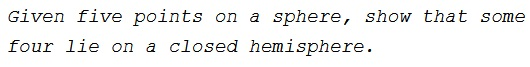# Problem A-2 from the 2002 Putnam Math Competition

### Problem### Solution 1

Draw a great circle through two of the points. There are two closed hemispheres with this great circle as boundary, and each of the other three points lies in one of them. By the pigeonhole principle, two of those three points lie in the same hemisphere, and that hemisphere thus contains four of the five given points.

Note: by a similar argument, one can prove that among any $n+3$ points on an $n-\text{dimensional}$ sphere, some $n+2$ of them lie on a closed hemisphere. (One cannot get by with only $n+2$ points: put them at the vertices of a regular simplex.) Namely, any $n$ of the points lie on a great sphere, which forms the boundary of two hemispheres; of the remaining three points, some two lie in the same hemisphere.

### Solution 2

Note first that of any four points on a circle, at least three are contained in some closed semicircle.

To turn to our assertion, let $P_1,\ldots,P_5$ be our points with $P_5=(0,0,1).$ By the remark above, there is a closed half-space that contains $P_5$ on its boundary and contains at least three of the other four points. More formally, let $P'_1,\ldots,P'_4$ be the points on the unit circle in the $xy-\text{plane},$ with center $O,$ such that the orthogonal projections of $P_i$ onto the $xy-\text{plane}$ is on the closed half-line $OP'_i.$ Let $H$ be a half-plane with $O$ on its boundary that contains at least three of the points $P'_1,\ldots,P'_4.$ Then the closed half-space determined by $H$ and $P_5$ contains four of our points.

### Acknowledgment

This is problem A-2 from the 2002 Putnam mathematical Competition. Solution 1 is by Kiran Kedlaya and Lenny Ng. Solution 2 is from The Art of Mathematics: Coffee Time in Memphis by B. Bollobás (Cambridge University Press, 2006).